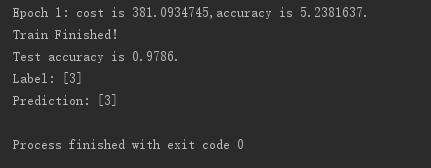## 一，多層感知機簡介

“多層”本質上指的是多層隱含層，神經網路的非線性也主要體現在隱含層非線性的啟用函式上，理論上只要隱含節點足夠多，只要有一層隱含層就可以擬合任意函式，但隱含層越多，就越容易擬合複雜函式，並且所需的隱含單元也越少。

• 過擬合
• 引數難以除錯
• 梯度消失
• 等等

1，Dropout解決過擬合問題

• 過擬合：神經網路的引數一般都很多，經常會出現引數比資料還要多的情況，所以過擬合很容易出現。
• Dropout：主要思想是在訓練時，將神經網路某些層的輸出節點資料隨機丟棄一部分，這種做法本質上等於創造了很多新的隨機的樣本，通過增大樣本量、減少特徵數量來防止過擬合。一般來說對越複雜越大的神經網路，dropout的效果越顯著。

2，自適應學習率演算法減輕除錯引數的負擔

• 引數難以除錯：主要是因為SGD的受學習速率的設定影響很大；神經網路通常不是一個凸優化問題，處處充分區域性最優；但有理論表明，神經網路可能有很多區域性最優解都可以達到比較好的分類效果，而全域性最優反而容易是過擬合的解。

3，Relu啟用函式解決梯度消失問題

• 梯度消失：當神經網路層數較多時，一些啟用函式如sigmoid啟用函式在誤差反向傳播中梯度值會逐漸減小，經過多層的傳遞後會呈指數級急劇減小，因此梯度值在傳遞到前面幾層時會變得非常小，小到無法更新神經網路的引數，起不到訓練的作用。
• Relu啟用函式：$y=max\left(0,x\right)$，類似人腦的閾值響應機制，由於右側是線性的，梯度值不會變，所以不會出現梯度消失的問題。
特點有三：
①單側抑制。
②相對寬闊的興奮邊界。
③稀疏啟用性。
神經元編碼的工作方式具有稀疏性，即大腦同時被啟用的神經元只有1%~4%，而sigmoid啟用函式會啟用幾乎一般的神經元嗎，不符合神經科學的研究。
Softplus雖然單側抑制，但是不具有稀疏啟用性，因而Relu及其變體(EIU、PRelu、RRelu、maxout等)成了最符合實際神經元也最流行的啟用函式，它們可以帶來訓練速度和模型準確率的提升，但是輸出層一般還是使用sigmoid或softmax啟用函式，因為它們最接近概率輸出分佈。

## 二，用TensorFlow實現MLP

• Dropout
• Relu啟用函式

• tf.nn.dropout(h1,keep_prob)
• tf.truncated_normal(shape,stddev)
• 之所以將W1初始化為截斷的正態分佈，是因為隱含層使用了Relu啟用函式，所以需要正態分佈給引數加一點噪聲，來打破完全對稱並且避免0梯度。在其他一些模型中，有時還需要給偏置賦上一些小的非零值來避免死亡神經元。而W2和b2直接賦值為0，是因為softmax啟用函式在0附近最敏感、梯度最大。

tensorflow的實現

# 多層感知機的實現
import tensorflow as tf
from tensorflow.examples.tutorials.mnist import input_data

# 0，匯入資料
print(mnist.train.images.shape,mnist.train.labels.shape)

# 1，定義模型計算公式
sess = tf.InteractiveSession()
in_units = 784
h1_units = 300
W1 = tf.Variable(tf.truncated_normal([in_units,h1_units],stddev=0.1))
b1 = tf.Variable(tf.zeros([h1_units]))
W2 = tf.Variable(tf.zeros([h1_units,10]))
b2 = tf.Variable(tf.zeros())

X = tf.placeholder(tf.float32,[None,in_units])
keep_prob = tf.placeholder(tf.float32,)
h1 = tf.nn.relu(tf.matmul(X,W1)+b1)
h1_drop = tf.nn.dropout(h1,keep_prob)
y_pred = tf.nn.softmax(tf.matmul(h1_drop,W2)+b2)

# 2,定義loss，選定優化器
y = tf.placeholder(tf.float32,[None,10])
cross_entropy = tf.reduce_mean(-tf.reduce_sum(y*tf.log(y_pred),reduction_indices=))

# 3,定義精確度計算公式
correct_prediction = tf.equal(tf.argmax(y_pred,1),tf.argmax(y,1))
accuracy = tf.reduce_mean(tf.cast(correct_prediction,tf.float32))

# 4,初始化引數
tf.global_variables_initializer().run()

# 4,迭代訓練
epoch_num = 1
for epoch in range(epoch_num):
avg_accuracy = 0.0
avg_cost = 0.0
for i in range(3000):
batch_xs,batch_ys = mnist.train.next_batch(100)
cost,acc,_ = sess.run([cross_entropy,accuracy,train_step],feed_dict={X:batch_xs,y:batch_ys,keep_prob:0.75})
avg_cost += cost
avg_accuracy += acc/3000
print('Epoch %d: cost is %.7f,accuracy is %.7f.'%(epoch+1,avg_cost,avg_accuracy))
print('Train Finished!')
print('Test accuracy is %.4f.'%accuracy.eval({X:mnist.test.images,y:mnist.test.labels,keep_prob:1.0}))

# 5,Get one and predict
import matplotlib.pyplot as plt
import random
r = random.randint(0, mnist.test.num_examples - 1)
print("Label:", sess.run(tf.argmax(mnist.test.labels[r:r+1], 1)))
print("Prediction:", sess.run(tf.argmax(y_pred, 1),feed_dict={X: mnist.test.images[r:r + 1],keep_prob:1.0}))
plt.imshow(mnist.test.images[r:r + 1].reshape(28, 28), cmap='Greys', interpolation='nearest')
plt.show()

sess.close()